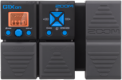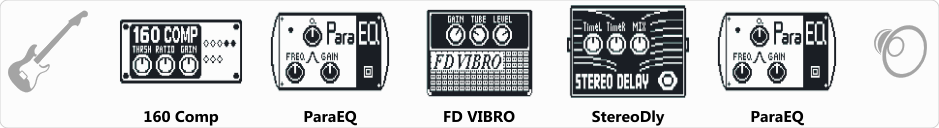# Klasz98

Discussion in 'Zoom G1on/G1Xon' started by Kovács László, Sep 17, 2018.Device: Zoom G1Xon
Firmware: 1.21

Optimized for: Phones/Speaker

Effects chain:I use this patch R&R solo too . Strait to PA.

Effect: "160 Comp" (Dynamics / Filter), active - "yes"
"THRSH" = -32
"Ratio" = 3.6
"Gain" = 0
"Knee" = Hard
"Level" = 103

Effect: "ParaEQ" (Dynamics / Filter), active - "yes"
"Freq1" = 1.0kHz
"Q1" = 1
"Gain1" = -5
"Freq2" = 3.2kHz
"Q2" = 1
"Gain2" = 11
"Level" = 76

Effect: "FD VIBRO" (Amp simulators), active - "yes"
"Gain" = 42
"Tube" = 27
"Level" = 142
"Trebl" = 10
"Middl" = 76
"Bass" = 39
"Prese" = 0
"CAB" = ALIEN 4x12
"OUT" = LINE

Effect: "StereoDly" (Delay / Reverb), active - "yes"
"TimeL" = 126
"TimeR" = 441
"Mix" = 58
"LchFB" = 20
"RchFB" = 54
"Level" = 114
"LchLv" = 62
"RchLv" = 100
"Tail" = Off

Effect: "ParaEQ" (Dynamics / Filter), active - "yes"
"Freq1" = 250Hz
"Q1" = 0.5
"Gain1" = 8
"Freq2" = 2.5kHz
"Q2" = 1
"Gain2" = 7
"Level" = 90
Patch Volume: 100

Note: This is a patch file, you will need to download and install the ToneLib software to use the patch.

#### Attached Files:

• ###### Klasz98.g1xon
File size:
432 bytes
Views:
113
Last edited: Oct 23, 2018
saobienorgan likes this.